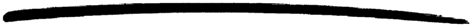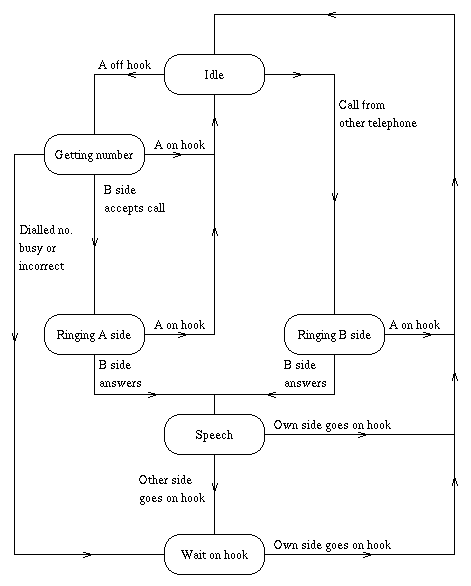## 6 Finite State Machines

This section describes the general principles of the Finite State Machine (FSM) behaviour and shows how to make FSM based applications. Refer to the Reference Manual `stdlib`, the module `gen_fsm` for additional information about this behaviour.

Many applications can be modeled as FSMs and be programmed using the `gen_fsm` behaviour. Protocol stacks are such an example.

A FSM can be described as a set of relations of the form:

```State(S) x Event(E) -> Actions (A), State(S')
...
```

These relations are interpreted as meaning:

If we are in state `S` and the event `E` occurs, we should perform the actions `A` and make a transition to the state `S'`.

If you program an FSM using the `gen_fsm` behaviour, then the state transition rules should be written as a number of Erlang functions which conform to the following convention:

```StateName(Event, StateData) ->
.. code for actions here ...
{next_state, StateName', StateData'}
```

The figure below is a simple FSM describing "Plain Ordinary Telephony Service" (POTS).FSM Example

The POTS FSM can be described by the following `gen_fsm` behaviour:

```init(A) ->
{ok, idle, A}.

idle({off_hook, A}, A) ->
{next_state, getting_number, {A,[]}};
idle({seize, A}, B) when A /= B ->
{next_state, ringing_b_side, {B, A});
idle(_, A) ->
{next_state, idle, A}.

getting_number({digit, D}, {A, Seq}) ->
case ... of
... ->
...
{next_state, ringing_a_side, {A, B}};
... ->
...
{next_state, getting_number, {A, Seq1}};
... ->
...
{next_state, wait_on_hook, A}
end;
getting_number({on_hook, A}, {A,_}) ->
{next_state, idle, A}.

ringing_a_side({on_hook, A}, {A, B}) ->
{next_state, idle, A};
{next_state, speech, {A,B}}.

ringing_b_side({on_hook, A}, {B, A}) ->
{next_state, idle, B};
ringing_b_side({off_hook, B}, {B, A}) ->
{next_state, speech, {B, A}}.

speech({on_hook, A}, {A, B}) ->
{next_state, idle, A};
speech({on_hook, B}, {A, B}) ->
{next_state, wait_on_hook, A}.

wait_on_hook({on_hook, A}, A) ->
{next_state, idle, A}.
```

The code shown above only describes the state transitions. To add the actions, we might add the following code:

```getting_number({digit, D}, {A, Seq}) ->
Seq1 = Seq ++ [D],
case number_analyser:analyse(Seq1) of
{user, B} ->
hw:seize(B, A),
{next_state, ringing_a_side, {A, B}};
get_more_digits ->
{next_state, getting_number, {A, Seq1}};
invalid_number ->
{next_state, wait_on_hook, A}
end;
getting_number({on_hook, A}, {A,_}) ->
hw:stop_codec(A),
{next_state, idle, A}.
```

To complete this example, we have to package the FSM and the event generation routines in a behaviour module, as shown in the following example, and then add the code for the FSM.

```-module(pots).
-behaviour(gen_fsm).
-export([...]).

start() -> gen_fsm:start(...)

stop() -> gen_fsm:send_all_state_event(...)

on_hook(A) -> gen_fsm:send_event(..., {off_hook, A}).
...
```

### 6.1 An FSM Example

The simple POTS example shown above does not include all required details. The following example is complete and hopefully also self explanatory.

```-module(test1_fsm).
-behaviour(gen_fsm).

%% interface routines

%% start us up
start() -> gen_fsm:start({local, hello}, test1_fsm, [], []).

%% send a hello -- this will end up in the FSM routines
hello() -> gen_fsm:send_event(hello, {hello, self()}).

%% send a stop this will end up in "handle_event"
stop()  -> gen_fsm:send_all_state_event(hello, stopit).

%% -- interface end

%% This initialisation routine gets called
init(_) ->
{ok, idle, []}.

%% The state machine
idle({hello, A}, []) ->
{next_state, one, A}.

one({hello, B}, A) ->
A ! {hello, B},
B ! {hello, A},
{next_state, idle, []}.

%% this handles events in *any* state
handle_event(stopit, AnyState, TheStateData) ->
{stop, i_shall_stop, []}.   %% tell it to stop

%% This is the finalisation routine - it gets called
%% When we have to stop
terminate(i_shall_stop, TheStateIwasIn, TheStateData) ->
ok.
```

### 6.2 Other Ways of Programming FSMs

This section has focused on the `gen_fsm` behaviour. A very large FSM, or an FSM which has a very regular structure, can be built another way. It is possible to automatically generate the code which describes the machine from the FSM specification.